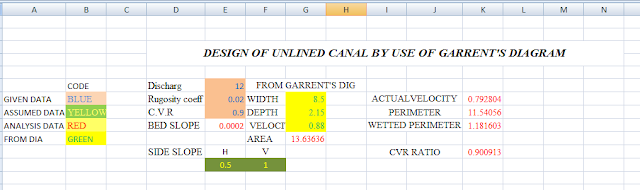# EXCEL SHEET OF DESIGN OF UNLINED CANAL BY GARRENT’S GRAPH

## HOW TO USE EXCEL SHEET OF DESIGN OF UNLINED CANAL BY GARRENT’S GRAPHEXCEL SHEET OF DESIGN OF UNLINED CANAL BY GARENNT,S GRAPH

First fall all data have colour code
1. Assumed data Yellow Colour
2. Given data in Blue Colour
3. Analysis data in Red Colour
4. Data from graph Green Colour
• first put all given data in excel sheet.
• Then take base on discharge and slope you get velocity, depth, and width.
• This data of graph put in excel sheet.
• Then automatically you get
• 1.      Area,
• 2.      Perimeter,
• 3.      Hydraulic Mean Depth
• 4.      Actual Velocity
• 5.      Velocity Ratio.
Then compare analysis velocity ratio to given critical velocity ratio. When the ratios are same then your design completed and you got perfect value of width and depth of irrigation canal.

If this ratios value are not same then change the graph data (like depth, velocity, and width of the canal). And repeat this procedure, till than you not get equal ratio of velocity.

NOTE :- IF YOU NOT KNOW HOW TO USE GARRENT’S GRAPH FOR DESIGN UNLINED CANAL SEE